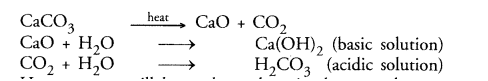# On heating, calcium carbonate gets`
Question:

On heating, calcium carbonate gets converted into calcium oxide and carbon dioxide.

1. Is this a physical or a chemical change ?

2. Can you prepare one acidic and one basic solution by using the products formed in the above process ? If so, write the chemical equation involved.

Solution:

1. It is chemical change. The chemical equation for the reaction is :

Calcium carbonate $\stackrel{\text { heat }}{\longrightarrow}$ Calcium oxide + Carbon dioxide

2. By adding water to calcium oxide taken in a test tube, a basic solution results. It contains calcium

hydroxide

Calcium oxide + Water $\rightarrow>$ Calcium hydroxide

By passing carbon dioxide through water taken in a tube, carbonic acid is formed. The solution is of

acidic nature

Carbon dioxide + Water $\longrightarrow \rightarrow$ Carbonic acid

Note:

All the three chemical equations are word equations. Symbol equations can also be written for these. For example,However, you will learn about these in the next class.